Details: ROBUSTREG Procedure

This section describes the statistical and computational aspects of the ROBUSTREG procedure. The following notation is used throughout this section.

Let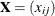denote an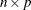matrix,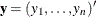denote a given n-vector of responses, and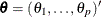denote an unknown p-vector of parameters or coefficients whose components are to be estimated. The matrixis called the design matrix. Consider the usual linear model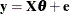where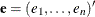is an n-vector of unknown errors. It is assumed that (for a given) the components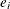ofare independent and identically distributed according to a distribution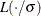, whereis a scale parameter (usually unknown). Often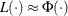, the standard normal distribution function. The vector of residuals for a given value of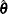is denoted by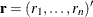and the ith row of the matrixis denoted by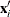.